Ex 6.1

Chapter 6 Class 6 Integers
Serial order wise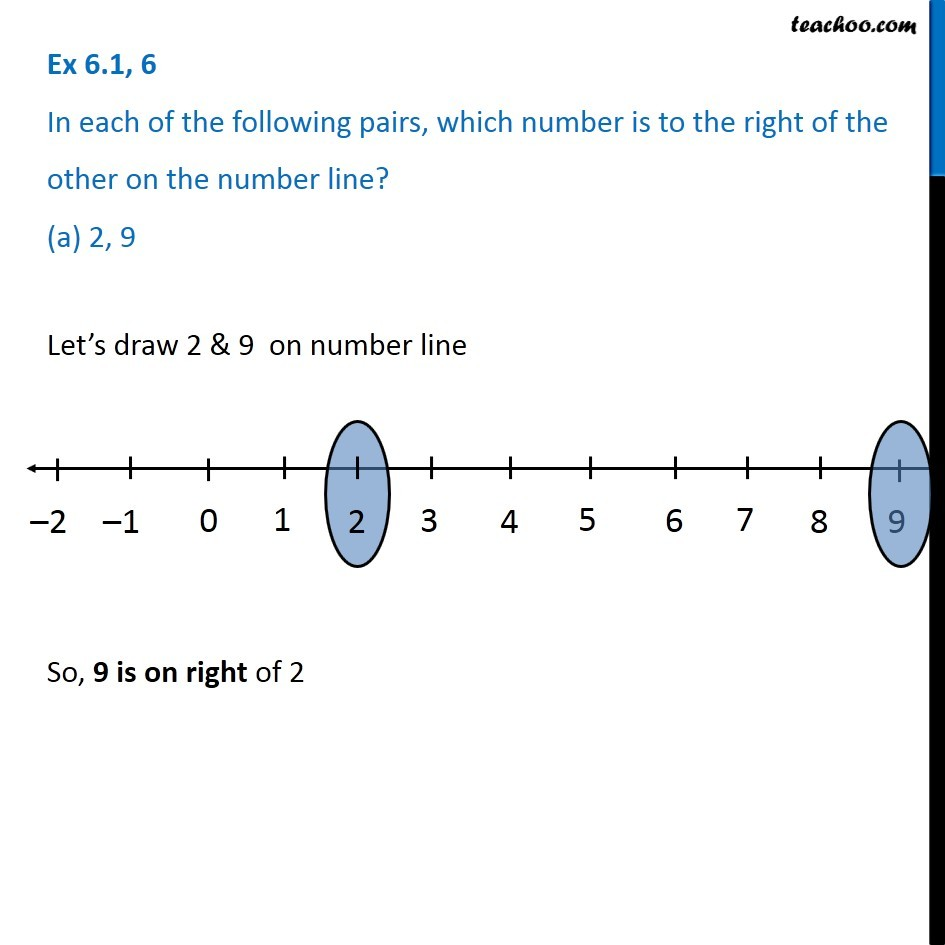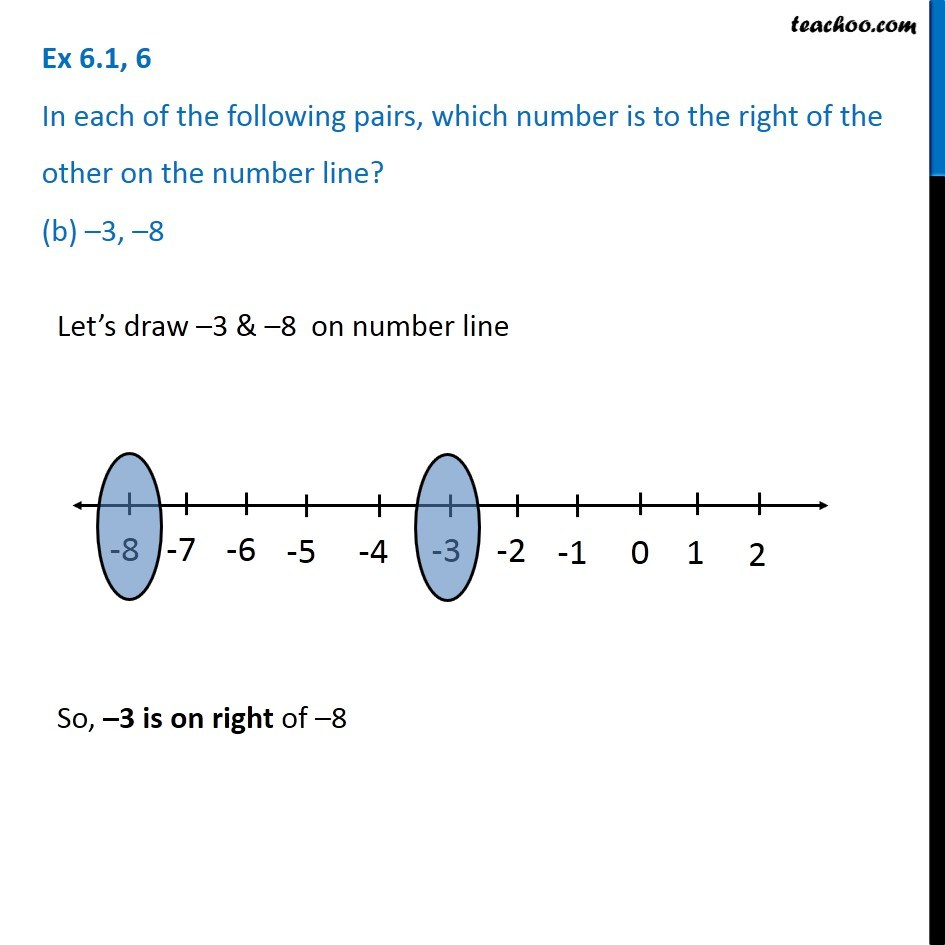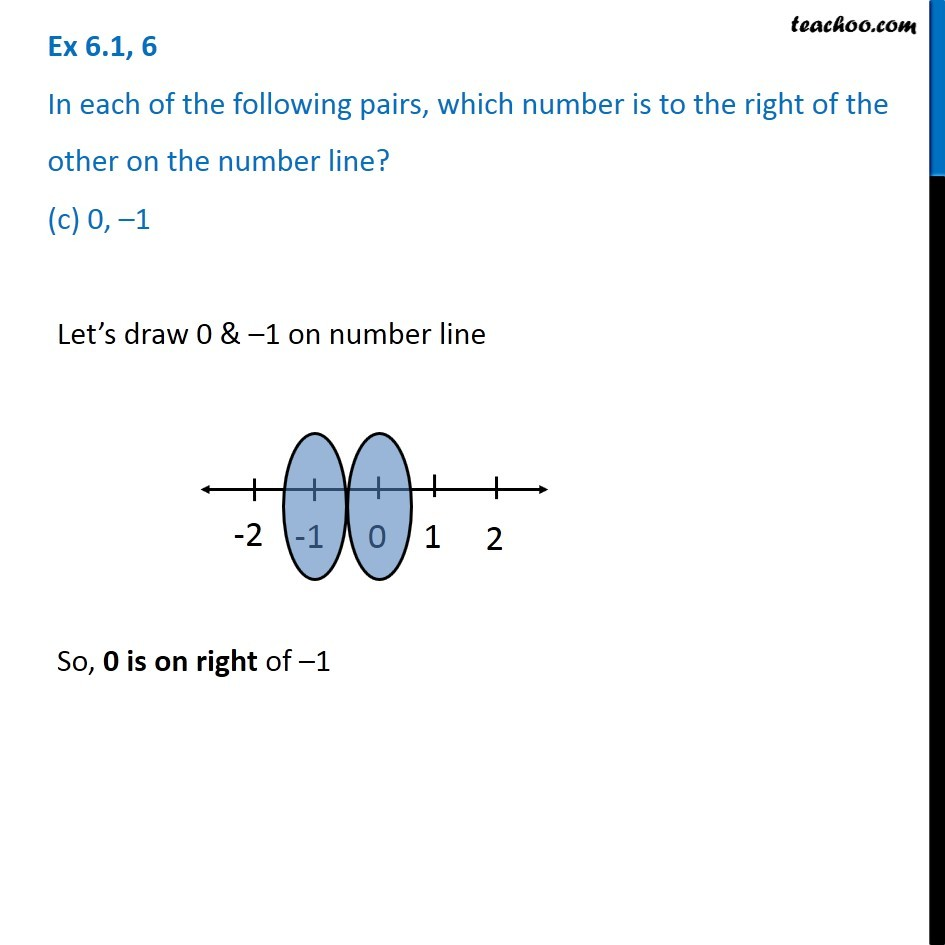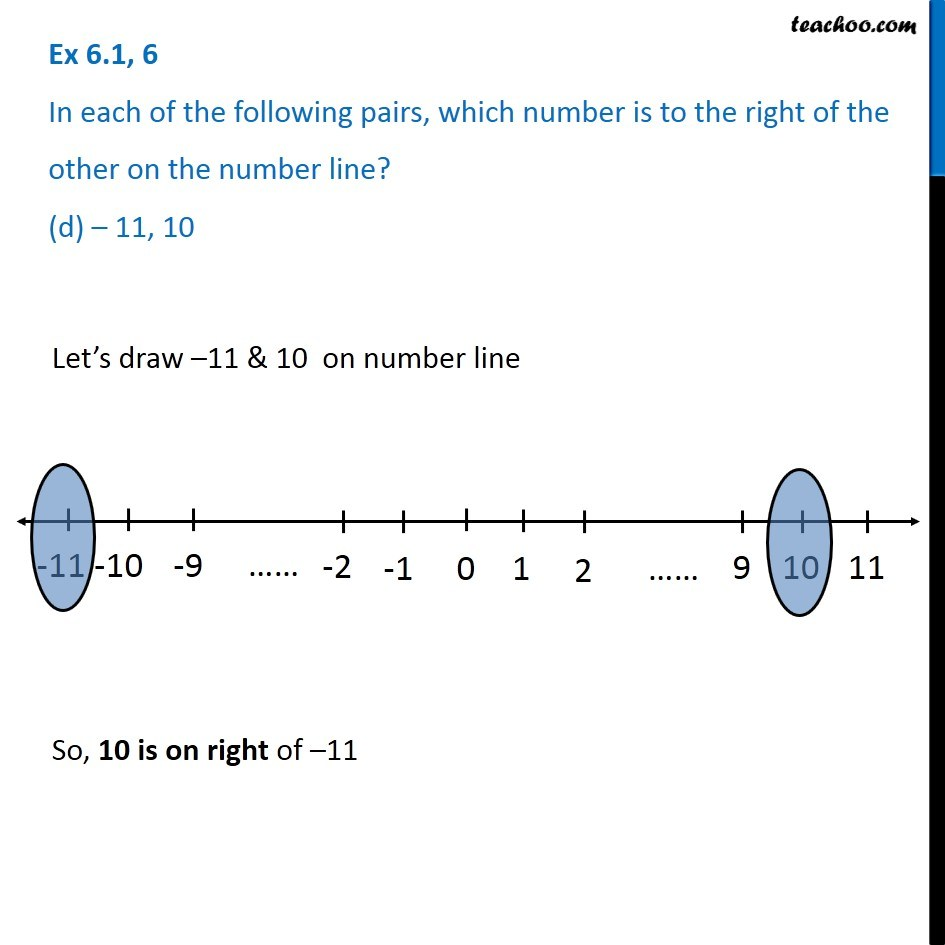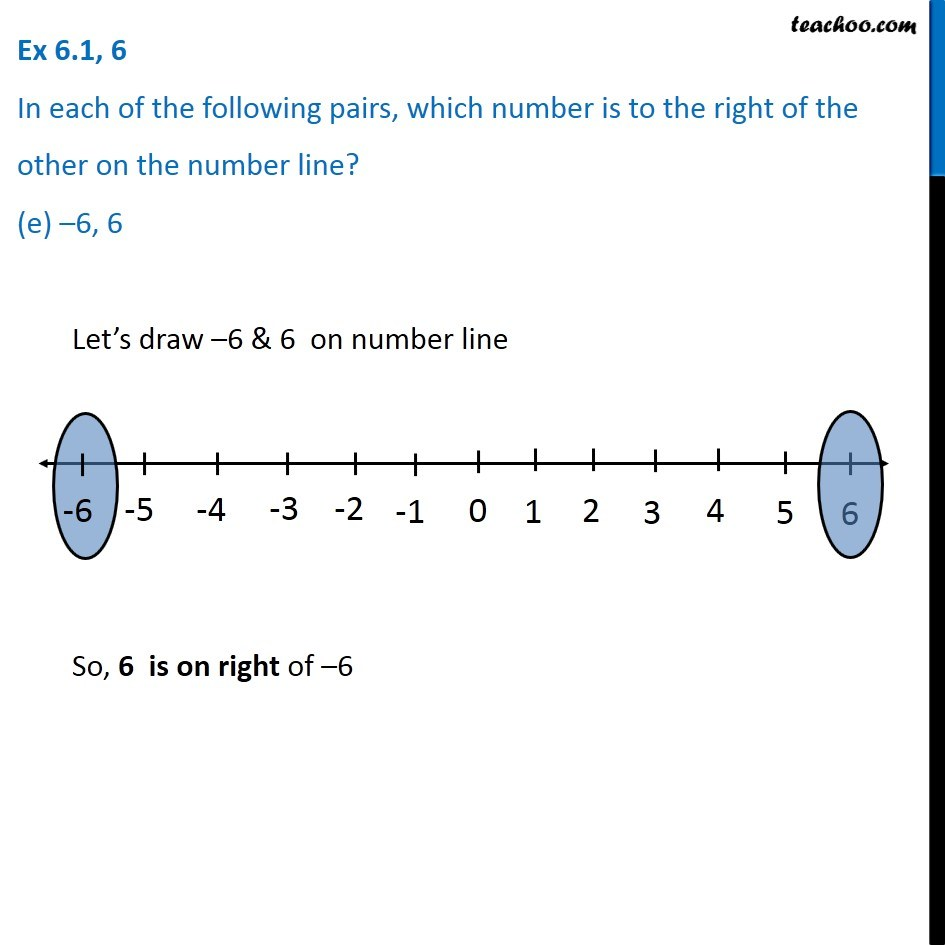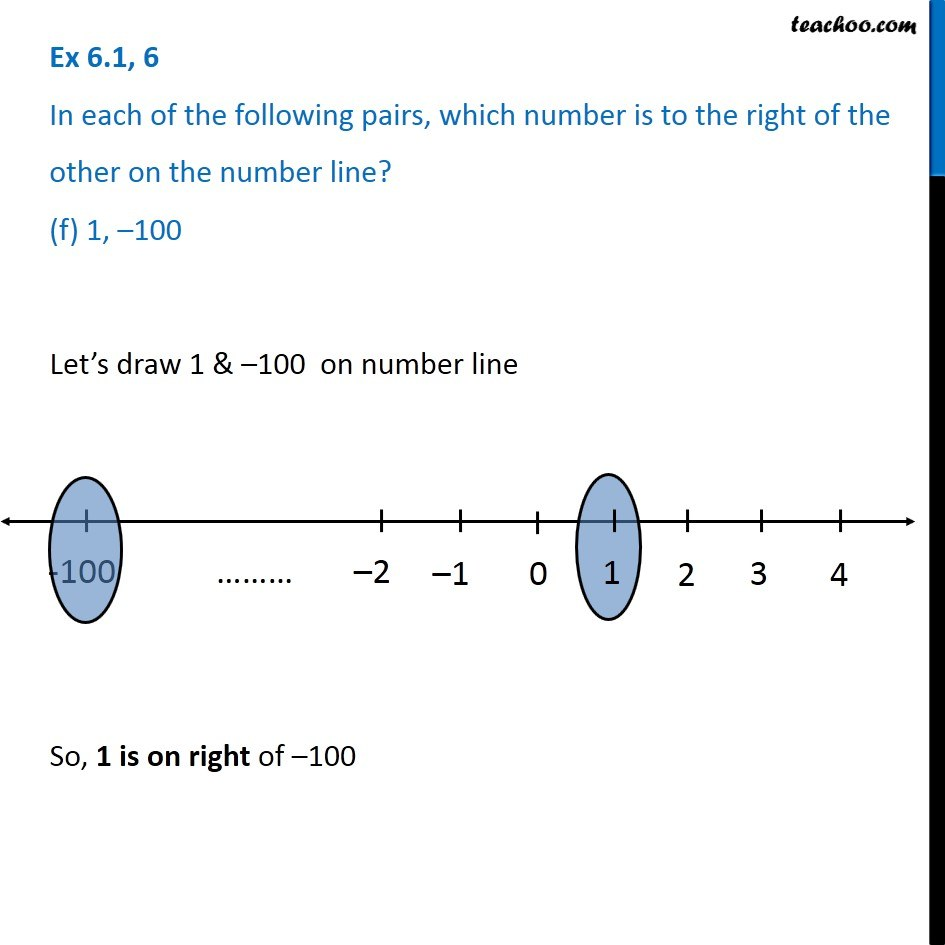### Transcript

Ex 6.1, 6 In each of the following pairs, which number is to the right of the other on the number line? (a) 2, 9Let’s draw 2 & 9 on number line So, 9 is on right of 2 Ex 6.1, 6 In each of the following pairs, which number is to the right of the other on the number line? (b) –3, –8Let’s draw –3 & –8 on number line So, –3 is on right of –8 Ex 6.1, 6 In each of the following pairs, which number is to the right of the other on the number line? (c) 0, –1Let’s draw 0 & –1 on number line So, 0 is on right of –1 Ex 6.1, 6 In each of the following pairs, which number is to the right of the other on the number line? (d) – 11, 10Let’s draw –11 & 10 on number line So, 10 is on right of –11 Ex 6.1, 6 In each of the following pairs, which number is to the right of the other on the number line? (e) –6, 6Let’s draw –6 & 6 on number line So, 6 is on right of –6 Ex 6.1, 6 In each of the following pairs, which number is to the right of the other on the number line? (f) 1, –100 Let’s draw 1 & –100 on number line So, 1 is on right of –100